# Probability and Statistics Notes | Study Topic wise GATE Past Year Papers for Civil Engineering - Civil Engineering (CE)

## Civil Engineering (CE): Probability and Statistics Notes | Study Topic wise GATE Past Year Papers for Civil Engineering - Civil Engineering (CE)

The document Probability and Statistics Notes | Study Topic wise GATE Past Year Papers for Civil Engineering - Civil Engineering (CE) is a part of the Civil Engineering (CE) Course Topic wise GATE Past Year Papers for Civil Engineering.
All you need of Civil Engineering (CE) at this link: Civil Engineering (CE)

Q. 1 The probability density function of a continuous random variable distributed uniformly between x and y (for y > x) is    [2019 : 2 Marks, Set-II]

(a) y - x
(b)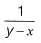(c) ) x - y

(d)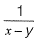Ans:  (b)

Probability density function of uniform distribution is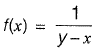Q. 2 Probability (up to one decimal place) of consecutively picking 3 red balls without replacement from a box containing 5 red balls and 1 white ball is _______ .    [2018 : 1 Mark, Set-II]
Ans (0.5)

Probability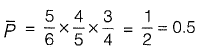Q. 3  The graph of a funotion f{x) is shown in the figure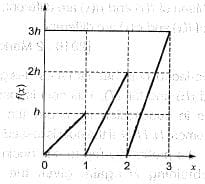For f(x) to be valid probability density function, the value of h is

[2018 : 1 Mark, Set-II]
(a) 1/3
(b) 2/3
(c)
(d) 3

Ans (a)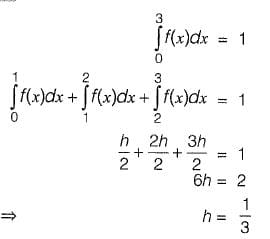Q. 4 A probability distribution with right skew is shown in the figure.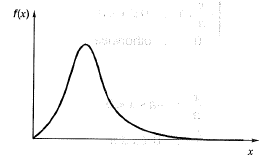The correct statement for the probability distribution is
(a) Mean is equal to mode
(b) Mean is greater than median but less than mode
(c) Mean is greater than median and mode
(d) Mode is greater than median.          [2018 :1 Mark, Set-II]

Ans (c)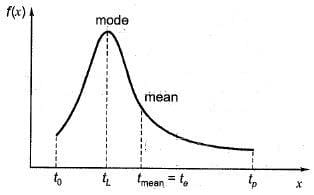tL < fmea = Curve is skew to right,
mode < mean
i.e., mean > median and mode
Mean is greater than the mode and the median. This is common for a distribution that is skewed, to the right [i.e., bunched up toward the left and a ‘tail’ stretching toward the right].

Q. 5 For the function f(x) = a + bx, 0 ≤ x ≤ 1, to be a valid probability density function, which one of the following statements is correct?
(a) a = 1, b = 4
(b) a = 0.5, b = 1
(c) a = 0, b = 1
(d)a  = 1, b = 1               [2017 : 2 Marks, Set-I]

Ans: (b)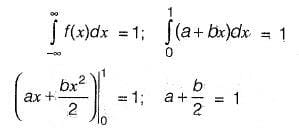Q. 6 The number of parameters in the univariate exponential and Gaussian distributions, respectively, are

(a) 2 and 2
(b) 1 and 2
(c) 2 and 1
(d) 1 and 1         [2017 : 1 Mark, Set-I]
Ans (b)

In exponential,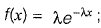x = 0

The parameter is λ.

In Gaussin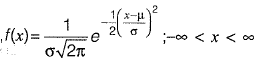The parameters are µ and α.

Q. 7 If f(x) arid y(x) are two probability density functions,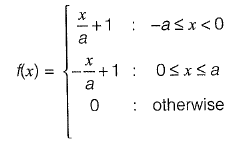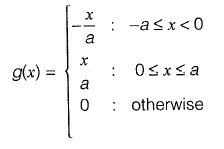Which one of the following statements is true?
(a) Mean of f(x) and g(x) are same; Variance of f{x) and g(x) are same

(b) Mean of f(x) and g(x) are same; Variance of f(x) and g(x) are different

(c) Mean of f(x) and g(x) are different; Variance of f(x) and g(x) are same

(d) Mean of f(x) and g(x) are different; Variance of f(x) and g(x) are different

Ans (b)

Mean of f(x) is t(x)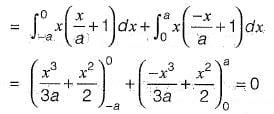Variance of f(x) is E(x)2 - {E(x)2} where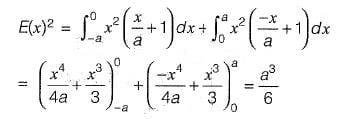⇒ Variance is a3/6

Next, mean of g(x) is E(x)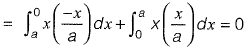Variance of g(x) is E(x2) - {E(x)}2, where,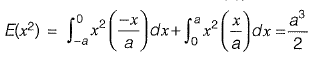⇒ Variance is a3/2

∴ Mean of f(x) and g(x) are same but variance of f(x) and g(x) are different

Q. 8 X and T are two random independent events. It is known that P (X ) = 0.40 and P { X ∪ Yc ) = 0.7. Which one of the following is the value of P (X ∪ Y)?    [2016 : 1 Mark, Set-II]
(a) 0.7
(b) 0.5
(c) 0.4
(d) 0.3

Ans (a)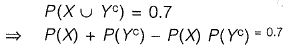(Since X, Y are independent events)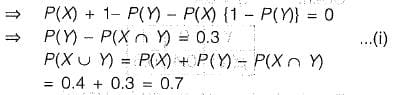Q. 9 Probability density function of a random variable X is given below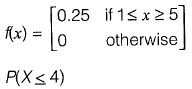[2016 : 2 Marks, Set-I]

(a) 3/4

(b) 1/4

(c) 1/4

(d) 1/8
Ans   (a)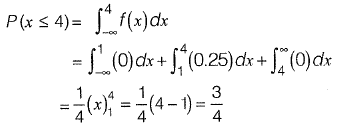Q. 10  The probability density function of a random variable, x is

Ans: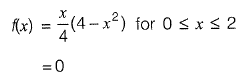The mean, μx of the random variable is________   [2015 : 2 Marks, Set-11]

Ans: 1.066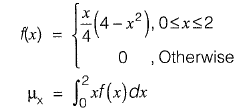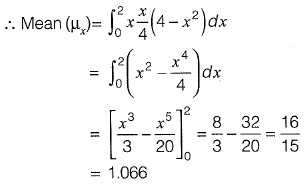Q. 11  Consider the following probability mass function (p.m.f.) of a random variable X.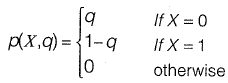if  q = 0.4, the variance of X is __________

Ans:  0.24

Given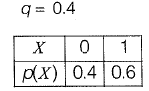Required value = V(X) = E{X2) - [E{X)]2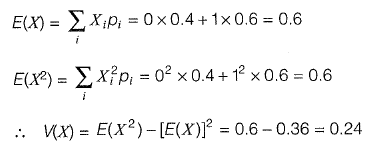Q. 12 A traffic office im poses on an average 5 number of penalties daily on traffic violators. Assume that the number of penalties on different days is independent and follows a Poisson distribution. The probability that there will be less than 4 penalties in a day is __________ .         [2014 : 2 Marks, Set-I

Ans: 0.265

Mean λ, = 5

P(x < 4) = { p(x = 0) + p(x = 1) + p(x = 2) + p(x = 3)}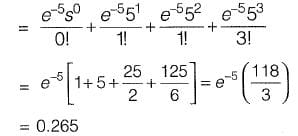Q. 13 A fair (unbiased) coin was tossed four times in succession and resulted in the following outcomes: (i) Head, (ii) Head, (iii) Head, (iv) Head. The probability of obtaining a 'Tail' when the coin is tossed again is

(a) 0
(b) 1/2
(c) 4/5
(d) 1/5
Ans: b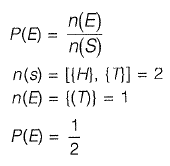Q. 14  The probability density function of evaporation E on any day during a year in a watershed is given by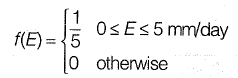The probability that Elies in between 2 and 4 mm/day in a day in the watershed is (in decimal)_____ .         [2014 : 1 Mark, Set-I]

Ans: 0.4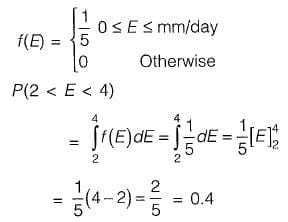Q. 15 Find the value of λ such that function f(x) is valid probability density function f(x) = λ(x - 1) ( 2 - x) for 1 ≤ x ≤ 2 = 0 otherwise    [2013 : 2 Marks]

Ans: 6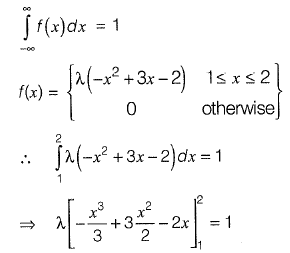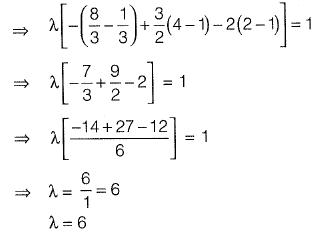Q. 17 In an experiment, positive and negative values are equally likely to occur. The probability of obtaining at most one negative value in five trials is (a) 1/32

(b) 2/32

(c) 3/32

(d) 6/32        [2011 : 2 Marks

Ans: d

Since negative and positive are equally likely, the distribution of number of negative values is binomial with n = 5 and p = 1/2
Let X represent number of negative values in 5 trials.
p(at most 1 negative value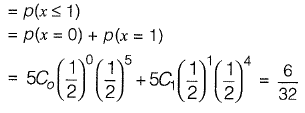Q. 18  The annual precipitation data of a city is normally distributed with mean and standard deviation as 1000 mm and 200 mm, respectively. The probability that the annual precipitation will be more than 1200 mm is
(a) <50%
(b) 50%
(c) 75%
(d) 100%   [2011 : 2 Marks]

Ans: a

The annual precipitation is normally distributed with μ = 1 000 mm and σ = 200 mm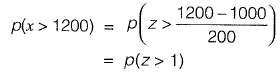Where z is the standard normal variate.

In normal distribution Now, since p(-1 < z < 1) ≈ 0.68

(≈ 68% of data is within one standard deviation of mean)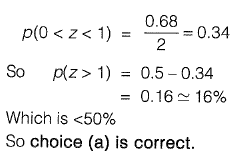Q. 19 There are two containers, with one containing 4 red and 3 green balls and the other containing 3 blue and 4 green balls. One ball is drawn at random from each container. The probability that one of the balls is red and the other is blue will be
(a) 1/7
(b) 9/49
(c) 12/49
(d) 3/7       [2011 : 1 Mark]
Ans: c

p(one ball is Red & another is blue)
= p(first is Red and second is Blue)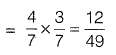Q. 20 Two coins are simultaneously tossed. The probability of two heads sim ultaneously appearing is       [2010 : 1 Mark]
(a) 1/8
(b) 1/6
(c) 1/4
(d) 1/2
Ans:  c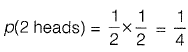The document Probability and Statistics Notes | Study Topic wise GATE Past Year Papers for Civil Engineering - Civil Engineering (CE) is a part of the Civil Engineering (CE) Course Topic wise GATE Past Year Papers for Civil Engineering.
All you need of Civil Engineering (CE) at this link: Civil Engineering (CE)Use Code STAYHOME200 and get INR 200 additional OFF

## Topic wise GATE Past Year Papers for Civil Engineering

67 docs

Track your progress, build streaks, highlight & save important lessons and more!

,

,

,

,

,

,

,

,

,

,

,

,

,

,

,

,

,

,

,

,

,

;